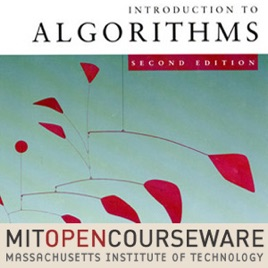23 episodios

This course teaches techniques for the design and analysis of efficient algorithms, emphasizing methods useful in practice. Topics covered include: sorting; search trees, heaps, and hashing; divide-and-conquer; dynamic programming; amortized analysis; graph algorithms; shortest paths; network flow; computational geometry; number-theoretic algorithms; polynomial and matrix calculations; caching; and parallel computing.# Introduction to Algorithms (2005) MIT

• Tecnología

This course teaches techniques for the design and analysis of efficient algorithms, emphasizing methods useful in practice. Topics covered include: sorting; search trees, heaps, and hashing; divide-and-conquer; dynamic programming; amortized analysis; graph algorithms; shortest paths; network flow; computational geometry; number-theoretic algorithms; polynomial and matrix calculations; caching; and parallel computing.

4.3 de 5
6 valoraciones

6 valoraciones